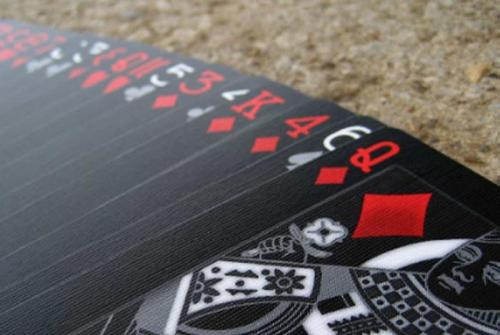# So Many Cards!

Probability Level 3Mursalin likes to collect playing-cards. In fact he has $1374$ decks of playing cards. One day he decides to take all of his decks and places them in a row on a (big!) table. He randomly takes out one card from the first deck and places it somewhere inside the second deck. Then he takes a random card out of the second deck and places it somewhere inside the third deck. He keeps on doing this with the other decks. He repeats the process until he takes out a random card from the $1374^{\text{th}}$ deck and there aren't any more decks left. The probability that the last card selected is an ace can be expressed as $\frac{a}{b}$ where $a$ and $b$ are co-prime positive integers. What is $a+b$?

Details and assumptions:

A regular deck of playing cards has $52$ cards $4$ of which are aces.

The image is taken from here.

×